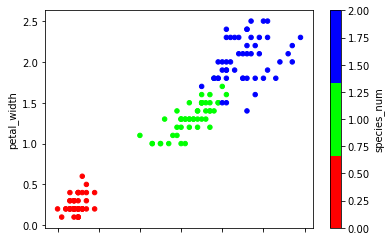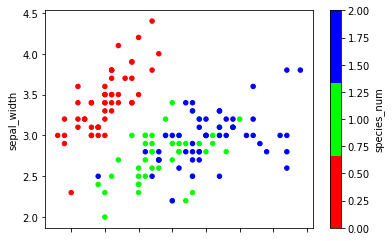# K-nearest neighbors and scikit-learn¶

## Agenda¶

1. Review of the iris dataset
2. Human learning on the iris dataset
3. K-nearest neighbors (KNN) classification
4. Review of supervised learning
5. Benefits and drawbacks of scikit-learn
6. Requirements for working with data in scikit-learn
7. scikit-learn's 4-step modeling pattern
8. Tuning a KNN model
9. Comparing KNN with other models

## Lesson goals¶

1. Learn how the modeling process works
2. Learn how scikit-learn works
3. Learn how KNN works

## Review of the iris dataset¶

In :
# read the iris data into a DataFrame
import pandas as pd
url = 'http://archive.ics.uci.edu/ml/machine-learning-databases/iris/iris.data'
col_names = ['sepal_length', 'sepal_width', 'petal_length', 'petal_width', 'species']

In :
iris.head()

Out:
sepal_length sepal_width petal_length petal_width species
0 5.1 3.5 1.4 0.2 Iris-setosa
1 4.9 3.0 1.4 0.2 Iris-setosa
2 4.7 3.2 1.3 0.2 Iris-setosa
3 4.6 3.1 1.5 0.2 Iris-setosa
4 5.0 3.6 1.4 0.2 Iris-setosa

### Terminology¶

• 150 observations (n=150): each observation is one iris flower
• 4 features (p=4): sepal length, sepal width, petal length, and petal width
• Response: iris species
• Classification problem since response is categorical

## Human learning on the iris dataset¶

How did we (as humans) predict the species of an iris?

1. We observed that the different species had (somewhat) dissimilar measurements.
2. We focused on features that seemed to correlate with the response.
3. We created a set of rules (using those features) to predict the species of an unknown iris.

We assumed that if an unknown iris has measurements similar to previous irises, then its species is most likely the same as those previous irises.

In :
# allow plots to appear in the notebook
%matplotlib inline
import matplotlib.pyplot as plt

# increase default figure and font sizes for easier viewing
plt.rcParams['figure.figsize'] = (6, 4)
plt.rcParams['font.size'] = 14

# create a custom colormap
from matplotlib.colors import ListedColormap
cmap_bold = ListedColormap(['#FF0000', '#00FF00', '#0000FF'])

In :
# map each iris species to a number
iris['species_num'] = iris.species.map({'Iris-setosa':0, 'Iris-versicolor':1, 'Iris-virginica':2})

In :
# create a scatter plot of PETAL LENGTH versus PETAL WIDTH and color by SPECIES
iris.plot(kind='scatter', x='petal_length', y='petal_width', c='species_num', colormap=cmap_bold)

Out:
<matplotlib.axes._subplots.AxesSubplot at 0x11c7744a8>In :
# create a scatter plot of SEPAL LENGTH versus SEPAL WIDTH and color by SPECIES
iris.plot(kind='scatter', x='sepal_length', y='sepal_width', c='species_num', colormap=cmap_bold)

Out:
<matplotlib.axes._subplots.AxesSubplot at 0x11c851940>## K-nearest neighbors (KNN) classification¶

1. Pick a value for K.
2. Search for the K observations in the data that are "nearest" to the measurements of the unknown iris.
• Euclidian distance is often used as the distance metric, but other metrics are allowed.
3. Use the most popular response value from the K "nearest neighbors" as the predicted response value for the unknown iris.

### KNN classification map for iris (K=1)¶### KNN classification map for iris (K=5)¶### KNN classification map for iris (K=15)¶### KNN classification map for iris (K=50)¶Question: What's the "best" value for K in this case?

Answer: The value which produces the most accurate predictions on unseen data. We want to create a model that generalizes!

## Review of supervised learning¶## Benefits and drawbacks of scikit-learn¶

Benefits:

• Consistent interface to machine learning models
• Provides many tuning parameters but with sensible defaults
• Exceptional documentation
• Rich set of functionality for companion tasks
• Active community for development and support

Potential drawbacks:

• Harder (than R) to get started with machine learning
• Less emphasis (than R) on model interpretability

Ben Lorica: Six reasons why I recommend scikit-learn

## Requirements for working with data in scikit-learn¶

1. Features and response should be separate objects
2. Features and response should be entirely numeric
3. Features and response should be NumPy arrays (or easily converted to NumPy arrays)
4. Features and response should have specific shapes (outlined below)
In :
iris.head()

Out:
sepal_length sepal_width petal_length petal_width species species_num
0 5.1 3.5 1.4 0.2 Iris-setosa 0
1 4.9 3.0 1.4 0.2 Iris-setosa 0
2 4.7 3.2 1.3 0.2 Iris-setosa 0
3 4.6 3.1 1.5 0.2 Iris-setosa 0
4 5.0 3.6 1.4 0.2 Iris-setosa 0
In :
# store feature matrix in "X"
feature_cols = ['sepal_length', 'sepal_width', 'petal_length', 'petal_width']
X = iris[feature_cols]

In :
# alternative ways to create "X"
X = iris.drop(['species', 'species_num'], axis=1)
X = iris.loc[:, 'sepal_length':'petal_width']
X = iris.iloc[:, 0:4]

In :
# store response vector in "y"
y = iris.species_num

In :
# check X's type
print(type(X))
print(type(X.values))

<class 'pandas.core.frame.DataFrame'>
<class 'numpy.ndarray'>

In :
# check y's type
print(type(y))
print(type(y.values))

<class 'pandas.core.series.Series'>
<class 'numpy.ndarray'>

In :
# check X's shape (n = number of observations, p = number of features)
print(X.shape)

(150, 4)

In :
# check y's shape (single dimension with length n)
print(y.shape)

(150,)


## scikit-learn's 4-step modeling pattern¶

Step 1: Import the class you plan to use

In :
from sklearn.neighbors import KNeighborsClassifier


Step 2: "Instantiate" the "estimator"

• "Estimator" is scikit-learn's term for "model"
• "Instantiate" means "make an instance of"
In :
# make an instance of a KNeighborsClassifier object
knn = KNeighborsClassifier(n_neighbors=1)
type(knn)

Out:
sklearn.neighbors.classification.KNeighborsClassifier
• Created an object that "knows" how to do K-nearest neighbors classification, and is just waiting for data
• Name of the object does not matter
• Can specify tuning parameters (aka "hyperparameters") during this step
• All parameters not specified are set to their defaults
In :
print(knn)

KNeighborsClassifier(algorithm='auto', leaf_size=30, metric='minkowski',
metric_params=None, n_jobs=1, n_neighbors=1, p=2,
weights='uniform')


Step 3: Fit the model with data (aka "model training")

• Model is "learning" the relationship between X and y in our "training data"
• Process through which learning occurs varies by model
• Occurs in-place
In :
knn.fit(X, y)

Out:
KNeighborsClassifier(algorithm='auto', leaf_size=30, metric='minkowski',
metric_params=None, n_jobs=1, n_neighbors=1, p=2,
weights='uniform')
• Once a model has been fit with data, it's called a "fitted model"

Step 4: Predict the response for a new observation

• New observations are called "out-of-sample" data
• Uses the information it learned during the model training process
In :
knn.predict([[3, 5, 4, 2]])

Out:
array()
• Returns a NumPy array, and we keep track of what the numbers "mean"
• Can predict for multiple observations at once
In :
X_new = [[3, 5, 4, 2], [5, 4, 3, 2]]
knn.predict(X_new)

Out:
array([2, 1])

## Tuning a KNN model¶

In :
# instantiate the model (using the value K=5)
knn = KNeighborsClassifier(n_neighbors=5)

# fit the model with data
knn.fit(X, y)

# predict the response for new observations
knn.predict(X_new)

Out:
array([1, 1])

Question: Which model produced the correct predictions for the two unknown irises?

Answer: We don't know, because these are out-of-sample observations, meaning that we don't know the true response values. Our goal with supervised learning is to build models that generalize to out-of-sample data. However, we can't truly measure how well our models will perform on out-of-sample data.

Question: Does that mean that we have to guess how well our models are likely to do?

Answer: Thankfully, no. In the next class, we'll discuss model evaluation procedures, which allow us to use our existing labeled data to estimate how well our models are likely to perform on out-of-sample data. These procedures will help us to tune our models and choose between different types of models.

In :
# calculate predicted probabilities of class membership
knn.predict_proba(X_new)

Out:
array([[0. , 0.8, 0.2],
[0. , 1. , 0. ]])